# Mathematics H110 Fall, 2003

CourseWeb H110 Web Page
Enrollment Information

## Professor Ken Ribet### 885 Evans Hall

Office: 510 642 0648
Fax: 510 642 8204
email: ribet@math.berkeley.edu

## Syllabus

Matrices, vector spaces, linear transformations, inner products, determinants. Eigenvectors. QF (sic.) factorization. Quadratic forms and Rayleigh's principle. Jordan canonical form, applications. Linear functionals.
This catalog description gives you some idea of what we might cover. If you look at the assignments for the course that I taught in 2002-2003, you'll get a sense of the set of topics that can be covered in a non-honors version of Math 110. We will certainly do things a bit differently in H110. In particular, the Jordan canonical form should be well within our reach.

## Textbook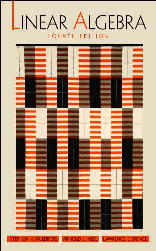Linear Algebra, 4th ed., by
Stephen H. Friedberg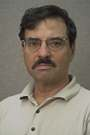, Arnold J. Insel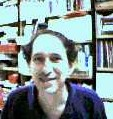, Lawrence E. Spence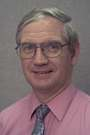.

Recommended Reading: There are quite a few good linear algebra books in circulation; see the textbook lists for some examples. Whenever you feel stuck when reading our text, feel free to consult alternative treatments. Reading several discussions of one topic is often illuminating.

One excellent book is Linear Algebra Done Right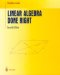by Sheldon Axler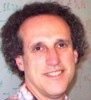. This book was based partly on ideas from Axler's article Down with Determinants!, which you might enjoy reading.

## Graduate Student Instructor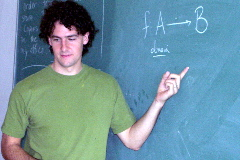The GSI for all Math 110 classes is Alexander J. Diesl. He will hold Math 110 office hours in 891 Evans as follows:
• Wednesday 10-12 AM and 1-4 PM
• Thursday 8-11 AM and 12-2 PM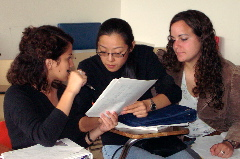## Examinations

• First Midterm: September 29, 2003, Questions and solution sketches.
Distribution of scores -- note the gap between 17 and 23: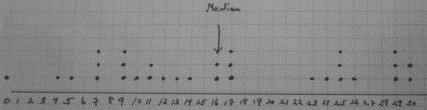• Second Midterm: November 3, 2003. Questions and solution sketches.
Distribution of scores -- sorry!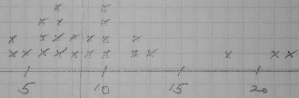• Final Exam: Tuesday, December 16, 2003, 5-8PM (Exam group 17) in 70 Evans. Questions and solution sketches.
Distribution of scores: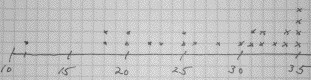Recall that students were asked to choose 6 out of the 8 problems. Despite indications to the contrary on the exam paper, all problems had equal weight: 6 points. The maximum score on the final was thus 36.
You might possibly find last year's tests useful in preparing for exams.

The H113 grading scheme for last semester worked out pretty well. (I think so, anyway!) I tried to clone it. The intention was to make the course components count as follows: Homework 25%, midterms 15% each, final 45%.

This class had 24 registered students (including one concurrent enrollment student); 23 students took the final exam. The grades for these students turned out as follows: 13 A's (including some A+ and A- grades), 9 B's, 1 C. See the grading table for more information.

## Homework Assignments

Homework will be assigned weekly. Because the Math 110 GSI will be available to answer your questions on Wednesday and Thursday of each week, most homework assignments will be due on Friday. When you prepare your homework, write carefully, in complete English sentences. Explain what you are doing, even if the problem is essentially a computation. Remember that the person grading the homework doesn't live inside your brain; when your homework is graded, your insights are represented only by the paper that you submitted. Please staple your assignment together before you submit it; we don't want pages to get lost!

See John Voight's Math H110 Web page for homework solutions.

1. Assignment due August 29: [mean = 16, standard deviation = 3]
• § 1.1:    7
• § 1.2:    1 (all parts), 8, 10, 16, 21
• § 1.3:    1 (all parts), 2h, 8 (all parts), 11, 13, 19, 20, 23
2. Assignment due September 5: [mean = 18, standard deviation = 2]
• § 1.4:    12, 13, 14, 15, 16 ,17
• § 1.5:    7, 8, 9, 10, 11, 13, 14, 15, 18
3. Assignment due September 12: [mean = 16, standard deviation = 4]
• Let F be a finite field, i.e., a field with a finite number of elements. Find a formula for the number of bases of the vector space F^n (n-space over F). The number in question should depend in a simple way on n and the number of elements of F.
• Suppose that V is a vector space over F with basis S and that E is a subfield of F. (Think of E as a field contained in F; an example would be R inside C.) We may view F an an E-vector space in a natural way: the "scalars" in E act on the "vectors" in F by multiplication. Suppose that T is a basis of F as an E-vector space. Show that the products ts with s in S and t in T form a basis of V as an E-vector space.
• § 1.6:    7, 8, 12, 13, 20, 21, 24, 26, 29, 31, 32
4. Assignment due September 19: [mean = 15, standard deviation = 3]
• § 2.1:    13, 14, 17, 21, 26, 27, 31, 32, 35
• § 2.2:    12, 13
• § 2.3:    12, 15, 16, 17
• Suppose that X and W are subspaces of a vector space V. Assume that V is the internal direct sum of X and W; this means (1) that the intersection of X and W is {0} and (2) that every vector in V is a sum x+w with x in X and w in W. Consider the map from X to V/W that sends x in X to the coset x + W in V/W. Prove that this map is an isomorphism of vector spaces.
5. Assignment due September 26. [mean = 15, standard deviation = 6]
On the first line, ignore the reference to problem 10 of § 2.6. This problem appears on the last line as well; do it only once!
6. Assignment due October 3: [mean = 18, standard deviation = 2]
• § 3.2:    14, 18, 19, 21, 22 [Watch out for problem 18, which is stated incorrectly in the first printing of the fourth edition. See the errata for the first printing for a correction.]
• § 3.3:    10
• § 4.2:    5, 6, 28, 29
7. Assignment due October 10: [mean = 17, standard deviation = 3]
• § 4.3:    11, 12, 16, 17, 20, 21, 22, 23, 25
• Let n be an integer that is non-zero in F. (For example, if F is the field of integers mod a prime number p, this means that n is not a multiple of p.) Show that the identity matrix of size n cannot be written in the form AB-BA where A and B are square matrices of size n.
• In the previous problem, what can you say when n=0 in F?
[These last two problems were suggested by George Bergman. I've written up a comment.]
8. Assignment due October 17: [mean = 17, standard deviation = 3]
• Please start reading Keith Conrad's treatment of a recent article by Harm Derksen that appeared in the American Mathematical Monthly. I will lecture on Wednesday, and maybe also on Friday, from this text.
• Suppose that T is a linear operator on a finite-dimensional F-vector space V. Suppose that W is a subspace of V that is T-stable in the sense that T(w) lies in W for all w in W. Show that the formula v+W |-> T(v)+W can be used to define a linear transformation U from V/W to V/W. This is the linear map that T induces on V/W. Prove that the characteristic polynomial of T is the product of the following two polynomials: the characteristic polynomial of U and the characteristic polynomial of the restriction of T to W (thought of as a map from W to W).
• § 5.1:    17, 18, 20, 21
• § 5.2:    20, 21, 22, 23
9. Assignment due October 24. [mean = 18, standard deviation = 3]
10. Assignment due October 31. [mean = 12, standard deviation = 6]
To read about orthogonal polynomials on the Net, you can start with Chapter 22 of Abramowitz and Stegun or the orthogonal polynomials page of Eric Weisstein's World of Mathematics.
11. Assignment due November 7: [mean = 15, standard deviation = 6]
• § 6.2:    10, 12, 13, 14, 15, 16
• § 6.3:    9, 14
• § 6.4:    10, 13, 14
12. Assignment due November 14; I removed §6.5 problem 7 on November 13 and added a solution for problem 16 on November 18. [mean = 15, standard deviation = 6]
13. Assignment due November 21. In the first problem, the conditions are that for each *non-zero* x there's a y such that H(x,y) is non-zero and similarly for each non-zero y. You can read the short Web biography of James Joseph Sylvester if you're curious about the person behind the "invariance of inertia" theorem. Sylvester was apparently the first mathematician to use the term "matrix" with its current Math 110 meaning; this was in 1850. Oh yeah, there's also a biography of Marie Ennemond Camille Jordan that you can read at the same time!
14. Assignment due December 3, 2003 and further review problems.

## Anonymous Feedback

Please let me know what I'm doing right and what I'm doing wrong. Constructive feedback is always welcome; don't hesitate to propose changes. You might be inspired by the comments that were made by students in Math H113 last semester and by those that were made by the Math 250 students two years ago.

Update: the comment box is no longer active; school is out. You can read the comments that were submitted for this course during the semester.

In the course evaluation that was conducted a few weeks ago: the instructor received 2 5's, 14 6's, and 7 7's for an average score of 6.22. Some students thought that the course wasn't sufficiently challenging, but plenty reported that the course was very hard.